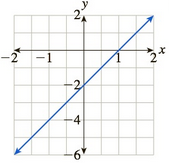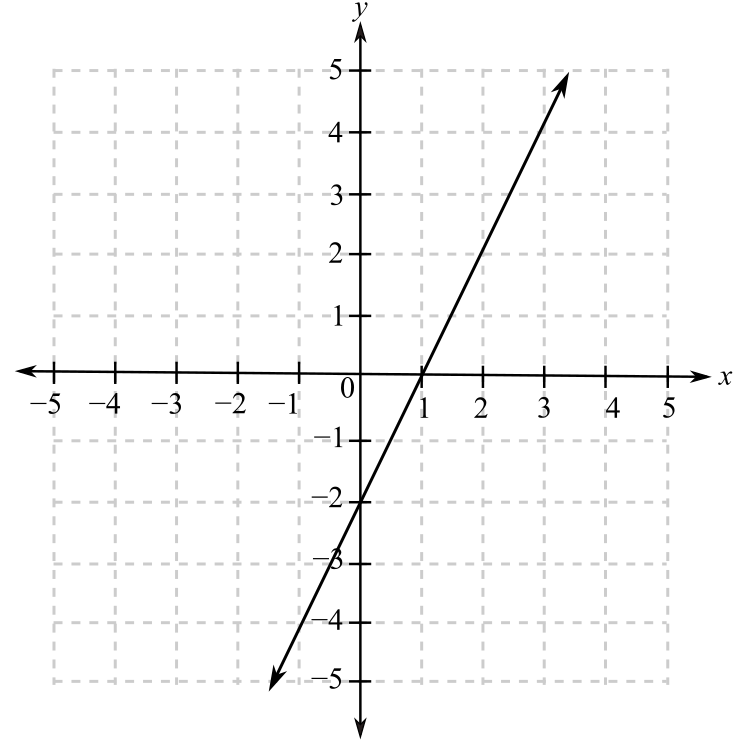Chapter 10.5, Problem 69EFinite Mathematics and Applied Cal...

7th Edition
Stefan Waner + 1 other
ISBN: 9781337274203

Solutions

Chapter
SectionFinite Mathematics and Applied Cal...

7th Edition
Stefan Waner + 1 other
ISBN: 9781337274203
Textbook Problem

In Exercises 69–72 the graph of the derivative of a function is given. For which x is the (original) function increasing? For which x is the (original) function decreasing? [HINT: See Quick Example 6.]To determine

The increasing and decreasing range of the function f from the graph of its derivative,Explanation

Given Information:

The provided graph is:

When the derivative of a function is positive in some interval then it is an increasing function in that interval and if the derivative is negative in some interval then it is a decreasing function in that interval.

From the graph, it can be observed that the derivative of the function is negative when x<1 and the der

Still sussing out bartleby?

Check out a sample textbook solution.

See a sample solution

The Solution to Your Study Problems

Bartleby provides explanations to thousands of textbook problems written by our experts, many with advanced degrees!

Get Started